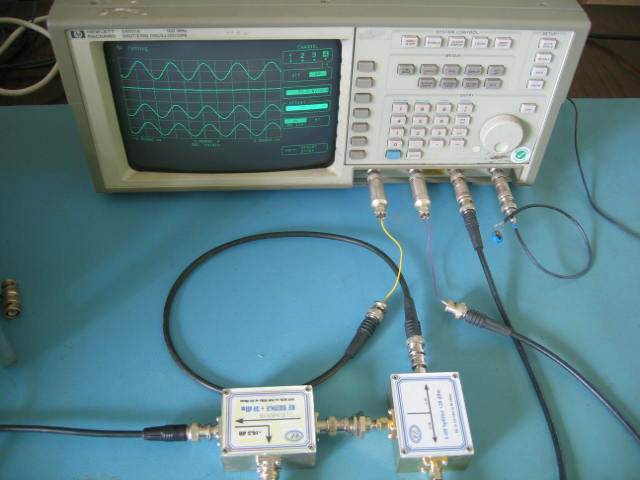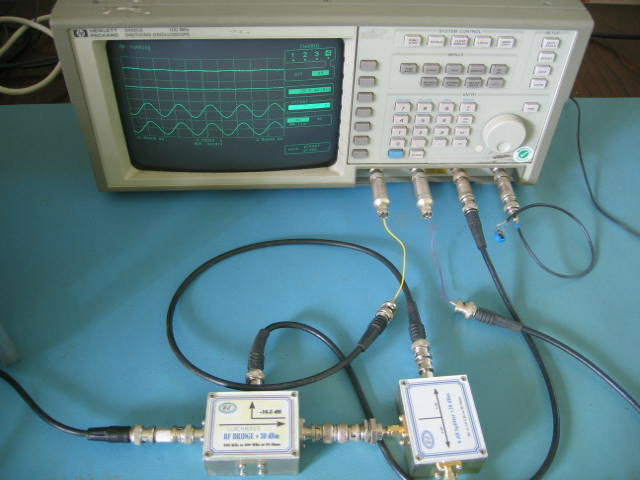# Antenna Current Distribution Page 2

In the process of measuring current distribution in an antenna, it is possible to use a toroidal transformer, a loop of wire or a current clamp. These methods all have one common characteristic, in as much they subtract INCIDENT and REFLECTED currents rather than add. This means that the results obtained from any measurements will mathematically be the Vector Difference of the INCIDENT and REFLECTED currents (or the vector sum with a negative sign for the reflected direction if you prefer it that way) and this measurement data would need processing if we wish to extract the independent INCIDENT and REFLECTED currents along the antenna. To make a directional current measurement in an antenna would be extremely difficult firstly because any form of directional coupler would have to be accurately matched to the antenna impedance at the specific measurement point and would also have to be moved along the length of the antenna. Secondly, in order to keep the length of the coupling device small, it would probably have to be a transformer type with screening and therefore grounded which would no doubt change the antenna performance so much that the results would not be representative of the normal behaviour of the antenna. Therefore, the use of an 'isolated' current transformer is the most practical way to measure antenna current.

Before starting the measurement and analysis of the current distribution it is essential that it is understood and accepted that the current transformer subtracts the INCIDENT and REFLECTED currents in the antenna. Proof of the fact that a toroidal transformer subtracts currents passing through it in opposite directions can be found by making measurements on two separate sample wires with known current direction and phase. The equipment is connected as per figure 4 and the signal generator set to a low frequency (in this case 1 MHz) in order to minimise problems caused by the screens of the sample wires being discontinuous and also to keep any phase variations to a minimum. Short cable lengths and short sample wires are required in order to avoid significant measurement errors caused by ground discontinuities. The 6 dB Splitter made by Chemandy Electronics is resistive and the phase difference between the two outputs is less than a degree at any frequency less than 1 GHz. The two short coaxial cables connected to the 6 dB splitter output are of the same length. The two short sample wires are also of the same length and just long enough to pass through the toroidal transformer in either direction. With the frequency set at 1 MHz, a difference in coaxial cable or sample wire length of 83 cm would give a phase error of one degree and therefore, the phase in the sample wires can be considered as being identical. All inputs to the oscilloscope are connected via 50 Ω terminations and ground continuity is maintained by the RF Bridge coupled output coax. The amplifier used is a 500 MHz in house version of the VHF 300 MHz amplifier.

Figure 4It can be seen from figure 5 that the top trace displays the INCIDENT signal at the coupled output of the bridge and that there is therefore, a signal current going towards the two sample wires. The second trace down shows the output from the toroidal transformer into a 50 Ohm load and this is not being used at this point. The two sample wire outputs are shown on the lower traces; displaying the voltage produced in the terminations by the two INCIDENT currents and showing that they are in phase.

Figure 5The bridge is now reversed and it can be seen from the top trace of figure 6 that there is virtually no signal at the coupled output of the coupler and that there is therefore, no significant REFLECTED current from the two sample wires or the splitter. We can therefore be sure that the currents in these sample wires substantially only flow in one direction, from the bridge towards the oscilloscope. The two sample wire outputs are again shown on the lower traces displaying the voltage produced in the terminations by the two INCIDENT currents and remain unchanged from figure 5.

Figure 61  2  3  4  5  6  7   >  Pages

How Does an Antenna Work Index

Technical Article Index